# Functions for Forward Mie Calculations of Homogeneous Spheres¶

## Functions for single particles¶

MieQ(m, wavelength, diameter[, nMedium=1.0, asDict=False, asCrossSection=False])

Computes Mie efficencies Q and asymmetry parameter g of a single, homogeneous particle. Uses Mie_ab() to calculate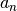and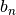, and then calculates Q via: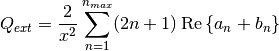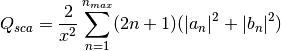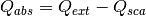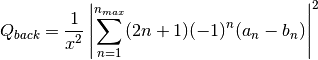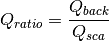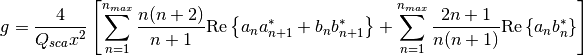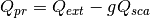where asterisks denote the complex conjugates.

Parameters

m : complex
The complex refractive index, with the convention m = n+ik.
wavelength : float
The wavelength of incident light, in nanometers.
diameter : float
The diameter of the particle, in nanometers.
nMedium : float, optional
The refractive index of the surrounding medium. This must be positive, nonzero, and real. Any imaginary part will be discarded.
asDict : bool, optional
If specified and set to True, returns the results as a dict.
asCrossSection : bool, optional
If specified and set to True, returns the results as optical cross-sections with units of nm2.

Returns

qext, qsca, qabs, g, qpr, qback, qratio : float
The Mie efficencies described above.
cext, csca, cabs, g, cpr, cback, cratio : float
If asCrossSection==True, MieQ() returns optical cross-sections with units of nm2.
q : dict
If asDict==True, MieQ() returns a dict of the above efficiencies with appropriate keys.
c : dict
If asDict==True and asCrossSection==True, returns a dict of the above cross-sections with appropriate keys.

For example, compute the Mie efficencies of a particle 300 nm in diameter with m = 1.77+0.63i, illuminated by λ = 375 nm:

>>> import PyMieScatt as ps
>>> ps.MieQ(1.77+0.63j,375,300,asDict=True)
{'Qext': 2.8584971991564112,
'Qsca': 1.3149276685170939,
'Qabs': 1.5435695306393173,
'g': 0.7251162362148782,
'Qpr': 1.9050217972664911,
'Qback': 0.20145510481352547,
'Qratio': 0.15320622543498222}

Mie_ab(m, x)

Computes external field coefficients an and bn based on inputs of m and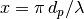. Must be explicitly imported via

>>> from PyMieScatt.Mie import Mie_ab


Parameters

m : complex
The complex refractive index with the convention m = n+ik.
x : float

The size parameter.

Returns

an, bn : numpy.ndarray
Arrays of size nmax = 2+x+4x1/3
Mie_cd(m, x)

Computes internal field coefficients cn and dn based on inputs of m and. Must be explicitly imported via

>>> from PyMieScatt.Mie import Mie_cd


Parameters

m : complex
The complex refractive index with the convention m = n+ik.
x : float

The size parameter.

Returns

cn, dn : numpy.ndarray
Arrays of size nmax = 2+x+4x1/3
RayleighMieQ(m, wavelength, diameter[, nMedium=1.0, asDict=False, asCrossSection=False])

Computes Mie efficencies of a spherical particle in the Rayleigh regime (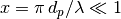) given refractive index m, wavelength, and diameter. Optionally returns the parameters as a dict when asDict is specified and set to True. Uses Rayleigh-regime approximations: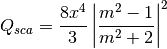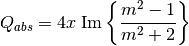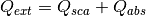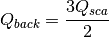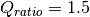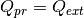Parameters

m : complex
The complex refractive index, with the convention m = n+ik.
wavelength : float
The wavelength of incident light, in nanometers.
diameter : float
The diameter of the particle, in nanometers.
nMedium : float, optional
The refractive index of the surrounding medium. This must be positive, nonzero, and real. Any imaginary part will be discarded.
asDict : bool, optional
If specified and set to True, returns the results as a dict.
asCrossSection : bool, optional
If specified and set to True, returns the results as optical cross-sections with units of nm2.

Returns

qext, qsca, qabs, g, qpr, qback, qratio : float
The Mie efficencies described above.
cext, csca, cabs, g, cpr, cback, cratio : float
If asCrossSection==True, RayleighMieQ() returns optical cross-sections.
q : dict
If asDict==True, RayleighMieQ() returns a dict of the above efficiencies with appropriate keys.
c : dict
If asDict==True and asCrossSection==True, returns a dict of the above cross-sections with appropriate keys.

For example, compute the Mie efficencies of a particle 50 nm in diameter with m = 1.33+0.01i, illuminated by λ = 870 nm:

>>> import PyMieScatt as ps
>>> ps.MieQ(1.33+0.01j,870,50,asDict=True)
{'Qabs': 0.004057286640269908,
'Qback': 0.00017708468873118297,
'Qext': 0.0041753430994240295,
'Qpr': 0.0041753430994240295,
'Qratio': 1.5,
'Qsca': 0.00011805645915412197,
'g': 0}

AutoMieQ(m, wavelength, diameter[, nMedium=1.0, crossover=0.01, asDict=False, asCrossSection=False])

Returns Mie efficencies of a spherical particle according to either MieQ() or RayleighMieQ() depending on the magnitude of the size parameter. Good for studying parameter ranges or size distributions. Thanks to John Kendrick for discussions about where to best place the crossover point.

Parameters

m : complex
The complex refractive index, with the convention m = n+ik.
wavelength : float
The wavelength of incident light, in nanometers.
diameter : float
The diameter of the particle, in nanometers.
nMedium : float, optional
The refractive index of the surrounding medium. This must be positive, nonzero, and real. Any imaginary part will be discarded.
crossover : float, optional
The size parameter that dictates where calculations switch from Rayleigh approximation to actual Mie.
asDict : bool, optional
If specified and set to True, returns the results as a dict.
asCrossSection : bool, optional
If specified and set to True, returns the results as optical cross-sections with units of nm2.

Returns

qext, qsca, qabs, g, qpr, qback, qratio : float
The Mie efficencies described above.
cext, csca, cabs, g, cpr, cback, cratio : float
If asCrossSection==True, AutoMieQ() returns optical cross-sections.
q : dict
If asDict==True, AutoMieQ() returns a dict of the above efficiencies with appropriate keys.
c : dict
If asDict==True and asCrossSection==True, returns a dict of the above cross-sections with appropriate keys.
LowFrequencyMieQ(m, wavelength, diameter[, nMedium=1.0, asDict=False, asCrossSection=False])

Returns Mie efficencies of a spherical particle in the low-frequency regime () given refractive index m, wavelength, and diameter. Optionally returns the parameters as a dict when asDict is specified and set to True. Uses LowFrequencyMie_ab() to calculate an and bn, and follows the same math as MieQ().

Parameters

m : complex
The complex refractive index, with the convention m = n+ik.
wavelength : float
The wavelength of incident light, in nanometers.
diameter : float
The diameter of the particle, in nanometers.
nMedium : float, optional
The refractive index of the surrounding medium. This must be positive, nonzero, and real. Any imaginary part will be discarded.
asDict : bool, optional
If specified and set to True, returns the results as a dict.
asCrossSection : bool, optional
If specified and set to True, returns the results as optical cross-sections with units of nm2.

Returns

qext, qsca, qabs, g, qpr, qback, qratio : float
The Mie efficencies described above.
cext, csca, cabs, g, cpr, cback, cratio : float
If asCrossSection==True, LowFrequencyMieQ() returns optical cross-sections.
q : dict
If asDict==True, LowFrequencyMieQ() returns a dict of the above efficiencies with appropriate keys.
c : dict
If asDict==True and asCrossSection==True, returns a dict of the above cross-sections with appropriate keys.

For example, compute the Mie efficencies of a particle 100 nm in diameter with m = 1.33+0.01i, illuminated by λ = 1600 nm:

>>> import PyMieScatt as ps
>>> ps.LowFrequencyMieQ(1.33+0.01j,1600,100,asDict=True)
{'Qabs': 0.0044765816617916582,
'Qback': 0.00024275862007727458,
'Qext': 0.0046412326004135135,
'Qpr': 0.0046400675577583459,
'Qratio': 1.4743834569616665,
'Qsca': 0.00016465093862185558,
'g': 0.0070758336692078412}

LowFrequencyMie_ab(m, x)

Returns external field coefficients an and bn based on inputs of m andby limiting the expansion of an and bn to second order: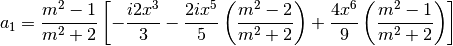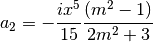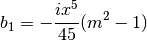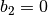Parameters

m : complex
The complex refractive index with the convention m = n+ik.
x : float

The size parameter.

Returns

an, bn : numpy.ndarray
Arrays of size 2.

## Functions for single particles across various ranges¶

MieQ_withDiameterRange(m, wavelength[, nMedium=1.0, diameterRange=(10, 1000), nd=1000, logD=False])

Computes the Mie efficencies of particles across a diameter range using AutoMieQ().

Parameters

m : complex
The complex refractive index with the convention m = n+ik.
wavelength : float
The wavelength of incident light, in nanomaters
nMedium : float, optional
The refractive index of the surrounding medium. This must be positive, nonzero, and real. Any imaginary part will be discarded.
diameterRange : tuple or list, optional
The diameter range, in nanometers. Convention is (smallest, largest). Defaults to (10, 1000).
nd : int, optional
The number of diameter bins in the range. Defaults to 1000.
logD : bool, optional
If True, will use logarithmically-spaced diameter bins. Defaults to False.

Returns

diameters : numpy.ndarray
An array of the diameter bins that calculations were performed on. Size is equal to nd.
qext, qsca, qabs, g, qpr, qback, qratio : numpy.ndarray
The Mie efficencies at each diameter in diameters.
MieQ_withWavelengthRange(m, diameter[, nMedium=1.0, wavelengthRange=(100, 1600), nw=1000, logW=False])

Computes the Mie efficencies of particles across a wavelength range using AutoMieQ(). This function can optionally take a list, tuple, or numpy.ndarray for m. If your particles have a wavelength-dependent refractive index, you can study it by specifying m as list-like. When doing so, m must be the same size as wavelengthRange, which is also specified as list-like in this situation. Otherwise, the function will construct a range from wavelengthRange to wavelengthRange with nw entries.

Parameters

m : complex or list-like
The complex refractive index with the convention m = n+ik. If dealing with a dispersive material, then len(m) must be equal to len(wavelengthRange).
diameter : float
The diameter of the particle, in nanometers.
nMedium : float, optional
The refractive index of the surrounding medium. This must be positive, nonzero, and real. Any imaginary part will be discarded. A future update will allow the entry of a spectral range of refractive indices.
wavelengthRange : tuple or list, optional
The wavelength range of incident light, in nanomaters. Convention is (smallest, largest). Defaults to (100, 1600). When m is list-like, len(wavelengthRange) must be equal to len(m).
nw : int, optional
The number of wavelength bins in the range. Defaults to 1000. This parameter is ignored if m is list-like.
logW : bool, optional
If True, will use logarithmically-spaced wavelength bins. Defaults to False. This parameter is ignored if m is list-like.

Returns

wavelengths : numpy.ndarray
An array of the wavelength bins that calculations were performed on. Size is equal to nw, unless m was list-like. Then wavelengths = wavelengthRange.
qext, qsca, qabs, g, qpr, qback, qratio : numpy.ndarray
The Mie efficencies at each wavelength in wavelengths.
MieQ_withSizeParameterRange(m[, nMedium=1.0, xRange=(1, 10), nx=1000, logX=False])

Computes the Mie efficencies of particles across a size parameter range () using AutoMieQ().

Parameters

m : complex
The complex refractive index with the convention m = n+ik.
nMedium : float, optional
The refractive index of the surrounding medium. This must be positive, nonzero, and real. Any imaginary part will be discarded.
xRange : tuple or list, optional
The size parameter range. Convention is (smallest, largest). Defaults to (1, 10).
nx : int, optional
The number of size parameter bins in the range. Defaults to 1000.
logX : bool, optional
If True, will use logarithmically-spaced size parameter bins. Defaults to False.

Returns

xValues : numpy.ndarray
An array of the size parameter bins that calculations were performed on. Size is equal to nx.
qext, qsca, qabs, g, qpr, qback, qratio : numpy.ndarray
The Mie efficencies at each size parameter in xValues.

## Functions for polydisperse size distributions of homogeneous spheres¶

When an efficiency Q is integrated over a size distribution nd(dp), the result is the coefficient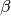, which carries units of inverse length. The general form is: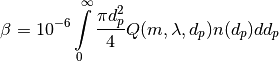where dp is the diameter of the particle (in nm), n(dp) is the number of particles of diameter dp (per cubic centimeter), and the factor 10-6 is used to cast the result in units of Mm-1.

The bulk asymmetry parameter G is calculated by: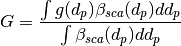Mie_SD(m, wavelength, sizeDistributionDiameterBins, sizeDistribution[, nMedium=1.0, asDict=False])

Returns Mie coefficients βext, βsca, βabs, G, βpr, βback, βratio. Uses scipy.integrate.trapz to compute the integral, which can introduce errors if your distribution is too sparse. Best used with a continuous, compactly-supported distribution.

Parameters

m : complex
The complex refractive index, with the convention m = n+ik.
wavelength : float
The wavelength of incident light, in nanometers.
sizeDistributionDiameterBins : list, tuple, or numpy.ndarray
The diameter bin midpoints of the size distribution, in nanometers.
sizeDistribution : list, tuple, or numpy.ndarray
The number concentrations of the size distribution bins. Must be the same size as sizeDistributionDiameterBins.
nMedium : float, optional
The refractive index of the surrounding medium. This must be positive, nonzero, and real. Any imaginary part will be discarded.
asDict : bool, optional
If specified and set to True, returns the results as a dict.

Returns

Bext, Bsca, Babs, G, Bpr, Bback, Bratio : float
The Mie coefficients calculated by AutoMieQ(), integrated over the size distribution.
q : dict
If asDict==True, MieQ_SD() returns a dict of the above values with appropriate keys.
Mie_Lognormal(m, wavelength, geoStdDev, geoMean, numberOfParticles[, nMedium=1.0, numberOfBins=1000, lower=1, upper=1000, gamma=, returnDistribution=False, decomposeMultimodal=False, asDict=False])

Returns Mie coefficients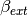,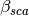,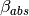,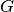,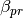,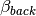, and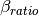, integrated over a mathematically-generated k-modal lognormal particle number distribution. Uses scipy.integrate.trapz to compute the integral.

The general form of a k-modal lognormal distribution is given by: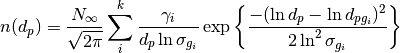where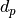is the diameter of the particle (in nm),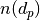is the number of particles of diameter(per cubic centimeter),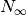is the total number of particles in the distribution,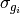is the geometric standard deviation of mode, and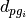is the geometric mean diameter (in nm) of the ith moment.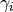is a porportionality constant that determines the fraction of total particles in the ith moment.

This function is essentially a wrapper for Mie_SD(). A warning will be raised if the distribution is not compactly-supported on the interval specified by lower and upper.

Parameters

m : complex
The complex refractive index, with the convention m = n+ik.
wavelength : float
The wavelength of incident light, in nanometers.
geoStdDev : float or list-like
The geometric standard deviation(s)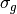orif list-like.
geoMean : float or list-like
The geometric mean diameter(s)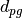orif list-like, in nanometers.
numberOfParticles : float
The total number of particles in the distribution.
nMedium : float, optional
The refractive index of the surrounding medium. This must be positive, nonzero, and real. Any imaginary part will be discarded.
numberOfBins : int, optional
The number of discrete bins in the distribution. Defaults to 1000.
lower : float, optional
The smallest diameter bin, in nanometers. Defaults to 1 nm.
upper : float, optional
The largest diameter bin, in nanometers. Defaults to 1000 nm.
gamma : list-like, optional
The porportionality coefficients for dividing total particles among modes.
returnDistribution : bool, optional
If True, both the size distribution bins and number concentrations will be returned.
decomposeMultimodal: bool, optional
If True (and returnDistribution==True), then the function returns an additional parameter containing the individual modes of the distribution.
asDict : bool, optional
If True, returns the results as a dict.

Returns

Bext, Bsca, Babs, G, Bpr, Bback, Bratio : float
The Mie coefficients calculated by MieQ(), integrated over the size distribution.
diameters, nd : numpy.ndarray
The diameter bins and number concentrations per bin, respectively. Only if returnDistribution is True.
ndi : list of numpy.ndarray objects
A list whose entries are the individual modes that created the multimodal distribution. Only returned if both returnDistribution and decomposeMultimodal are True.
B : dict
If asDict==True, MieQ_withLognormalDistribution() returns a dict of the above values with appropriate keys.

For example, compute the Mie coefficients of a lognormal size distribution with 1000000 particles, σg = 1.7, and dpg = 200 nm; with m = 1.60+0.08i and λ = 532 nm:

>>> import PyMieScatt as ps
>>> ps.MieQ_Lognormal(1.60+0.08j,532,1.7,200,1e6,asDict=True)
{'Babs': 33537.324569179938,
'Bback': 10188.473118449627,
'Bext': 123051.1109783932,
'Bpr': 62038.347528346232,
'Bratio': 12701.828124508347,
'Bsca': 89513.786409213266,
'bigG': 0.6816018615403715}


## Angular Functions¶

These functions compute the angle-dependent scattered field intensities and scattering matrix elements. They return arrays that are useful for plotting.

ScatteringFunction(m, wavelength, diameter[, nMedium=1.0, minAngle=0, maxAngle=180, angularResolution=0.5, space='theta', angleMeasure='radians', normalization=None])

Creates arrays for plotting the angular scattering intensity functions in theta-space with parallel, perpendicular, and unpolarized light. Also includes an array of the angles for each step. This angle can be in either degrees, radians, or gradians for some reason. The angles can either be geometrical angle or the qR vector (see Sorensen, M. Q-space analysis of scattering by particles: a review. J. Quant. Spectrosc. Radiat. Transfer 2013, 131, 3-12). Uses MieS1S2() to compute S1 and S2, then computes parallel, perpendicular, and unpolarized intensities by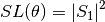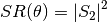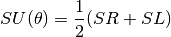Parameters

m : complex
The complex refractive index with the convention m = n+ik.
wavelength : float
The wavelength of incident light, in nanometers.
diameter : float
The diameter of the particle, in nanometers.
nMedium : float, optional
The refractive index of the surrounding medium. This must be positive, nonzero, and real. Any imaginary part will be discarded.
minAngle : float, optional
The minimum scattering angle (in degrees) to be calculated. Defaults to 0.
maxAngle : float, optional
The maximum scattering angle (in degrees) to be calculated. Defaults to 180.
angularResolution : float, optional
The resolution of the output. Defaults to 0.5, meaning a value will be calculated for every 0.5 degrees.
space : str, optional
The measure of scattering angle. Can be ‘theta’ or ‘qspace’. Defaults to ‘theta’.
angleMeasure : str, optional
The units for the scattering angle
normalization : str or None, optional

Specifies the normalization method, which is either by total signal or maximum signal.

• normalization = ‘t’ will normalize by the total integrated signal, that is, the total signal will have an integrated value of 1.
• normalization = ‘max’ will normalize by the maximum value of the signal regardless of the angle at which it occurs, that is, the maximum signal at that angle will have a value of 1.

Returns

theta : numpy.ndarray
An array of the angles used in calculations. Values will be spaced according to angularResolution, and the size of the array will be (maxAngle-minAngle)/angularResolution.
SL : numpy.ndarray
An array of the scattered intensity of left-polarized (perpendicular) light. Same size as the theta array.
SR : numpy.ndarray
An array of the scattered intensity of right-polarized (parallel) light. Same size as the theta array.
SU : numpy.ndarray
An array of the scattered intensity of unpolarized light, which is the average of SL and SR. Same size as the theta array.
SF_SD(m, wavelength, dp, ndp[, nMedium=1.0, minAngle=0, maxAngle=180, angularResolution=0.5, space='theta', angleMeasure='radians', normalization=None])

Creates arrays for plotting the angular scattering intensity functions in theta-space with parallel, perpendicular, and unpolarized light. Also includes an array of the angles for each step for a distribution nd(dp). Uses ScatteringFunction() to compute scattering for each particle size, then sums the contributions from each bin.

Parameters

m : complex
The complex refractive index with the convention m = n+ik.
wavelength : float
The wavelength of incident light, in nanometers.
dp : list-like
The diameter bins of the distribution, in nanometers.
ndp : list-like
The number of particles in each diameter bin in dp.
nMedium : float, optional
The refractive index of the surrounding medium. This must be positive, nonzero, and real. Any imaginary part will be discarded.
minAngle : float, optional
The minimum scattering angle (in degrees) to be calculated. Defaults to 0.
maxAngle : float, optional
The maximum scattering angle (in degrees) to be calculated. Defaults to 180.
angularResolution : float, optional
The resolution of the output. Defaults to 0.5, meaning a value will be calculated for every 0.5 degrees.
space : str, optional
The measure of scattering angle. Can be ‘theta’ or ‘qspace’. Defaults to ‘theta’.
angleMeasure : str, optional
The units for the scattering angle
normalization : str or None, optional

Specifies the normalization method, which is either by total particle number, total signal or maximum signal.

• normalization = ‘n’ will normalize by the total number of particles (the integral of the size distribution). Can lead to weird interpretations, so use caution.
• normalization = ‘t’ will normalize by the total integrated signal, that is, the total signal will have an integrated value of 1.
• normalization = ‘max’ will normalize by the maximum value of the signal regardless of the angle at which it occurs, that is, the maximum signal at that angle will have a value of 1.

Returns

theta : numpy.ndarray
An array of the angles used in calculations. Values will be spaced according to angularResolution, and the size of the array will be (maxAngle-minAngle)/angularResolution.
SL : numpy.ndarray
An array of the scattered intensity of left-polarized (perpendicular) light. Same size as the theta array.
SR : numpy.ndarray
An array of the scattered intensity of right-polarized (parallel) light. Same size as the theta array.
SU : numpy.ndarray
An array of the scattered intensity of unpolarized light, which is the average of SL and SR. Same size as the theta array.
MatrixElements(m, wavelength, diameter, mu[, nMedium=1.0])

Calculates the four nonzero scattering matrix elements S11, S12, S33, and S34 as functions of μ=cos(θ), where θ is the scattering angle: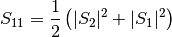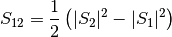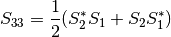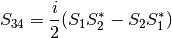Parameters

m : complex
The complex refractive index with the convention m = n+ik.
wavelength : float
The wavelength of incident light, in nanometers.
diameter : float
The diameter of the particle, in nanometers.
mu : float
The cosine of the scattering angle.
nMedium : float, optional
The refractive index of the surrounding medium. This must be positive, nonzero, and real. Any imaginary part will be discarded.

Returns

S11, S12, S33, S34 : float
The matrix elements described above.
MieS1S2(m, x, mu)

Calculates S1 and S2 at μ=cos(θ), where θ is the scattering angle.

Uses Mie_ab() to calculate an and bn, and MiePiTau() to calculate πn and τn. S1 and S2 are calculated by: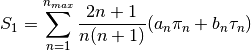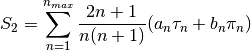Parameters

m : complex
The complex refractive index with the convention m = n+ik.
x : float
The size parameter.
mu : float
The cosine of the scattering angle.

Returns

S1, S2 : complex
The S1 and S2 values.
MiePiTau(mu, nmax)

Calculates πn and τn.

This function uses recurrence relations to calculate πn and τn, beginning with π0 = 1, π1 = 3μ (where μ is the cosine of the scattering angle), τ0 = μ, and τ1 = 3cos(2cos-1 (μ)):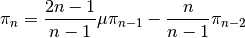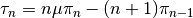Parameters

mu : float
The cosine of the scattering angle.
nmax : int
The number of elements to compute. Typically, nmax = floor(2+x+4x1/3), but can be given any integer.

Returns

p, t : numpy.ndarray
The πn and τn arrays, of length nmax.